# How to detect 3D video?

by | | Read ~430 times.

Here's an interesting conundrum. My TV can automatically detect when 3D video is being played and offers to switch into 3D mode - but how does the detection work?

This post will give you a few strategies for detecting 3D images using Python.

Firstly, some terminology.

3D videos are usually saved either as Side-By-Side images, or Over-Under images. Colloquially known as H-SBS and H-OU. Here's an example.

### SBS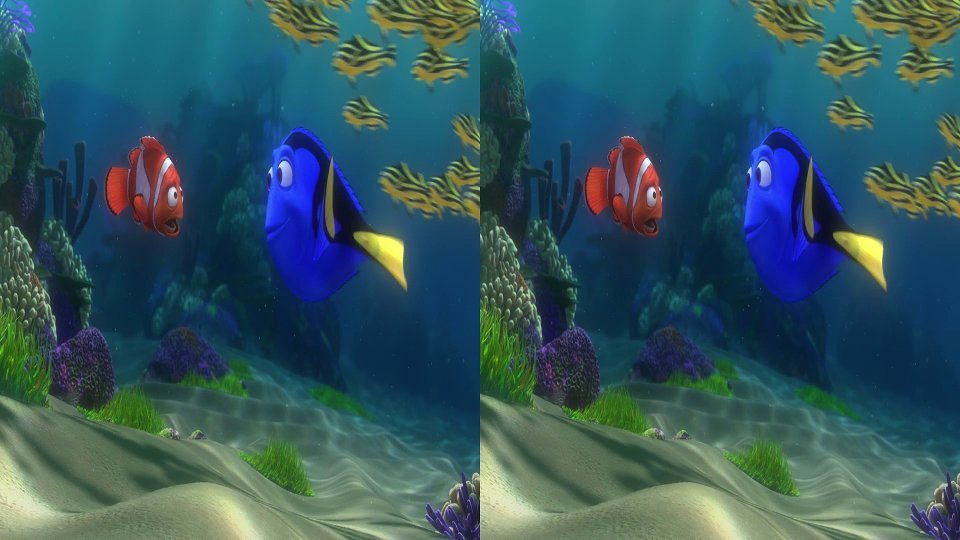### OU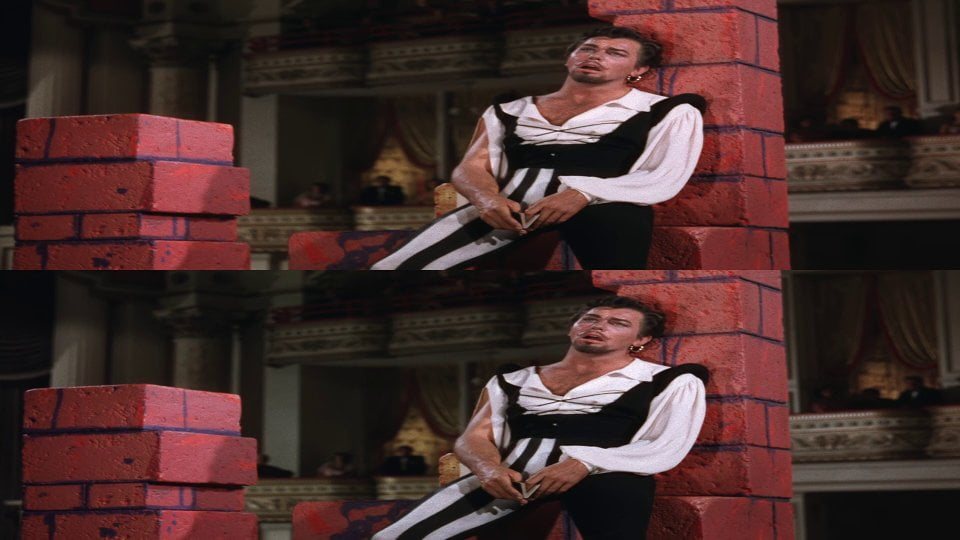My first thought was that 3D video may contain metadata which the TV picks up on. This is not the case. If I display a still image from a USB stick, my TV offers to convert it. Additionally, the TV sometimes makes mistakes! If it sees a scene which has substantially similar content on each side, it will offer to convert it.

## Detecting the split

There's a very obvious horizontal or vertical line in the images. It's an optical illusion caused by graphical differences between the two sides. A computer can see this line using edge detection.

``````from PIL import Image
from PIL import ImageFilter
Image.open("Finding_Nemo_01.png").convert("L").filter(ImageFilter.FIND_EDGES).show()
``````

Here are the edge detected versions.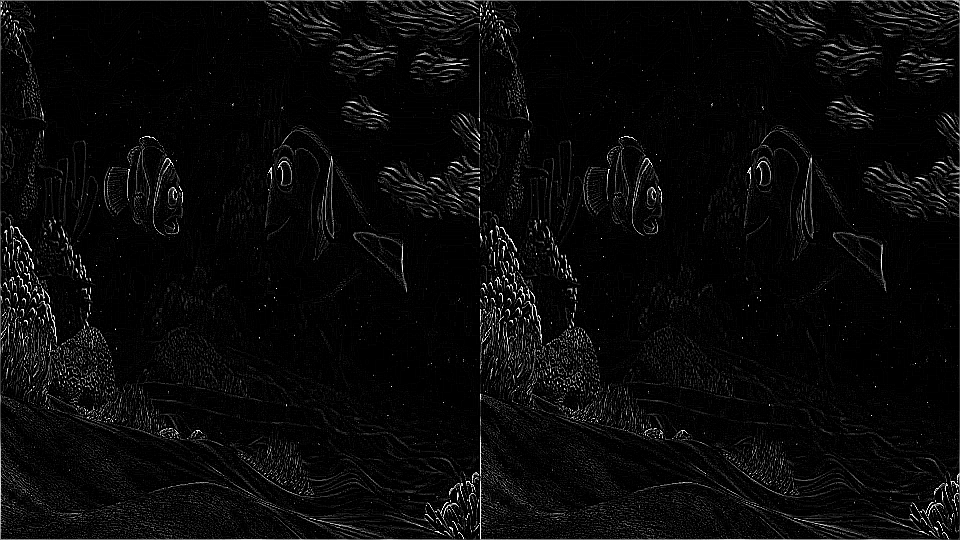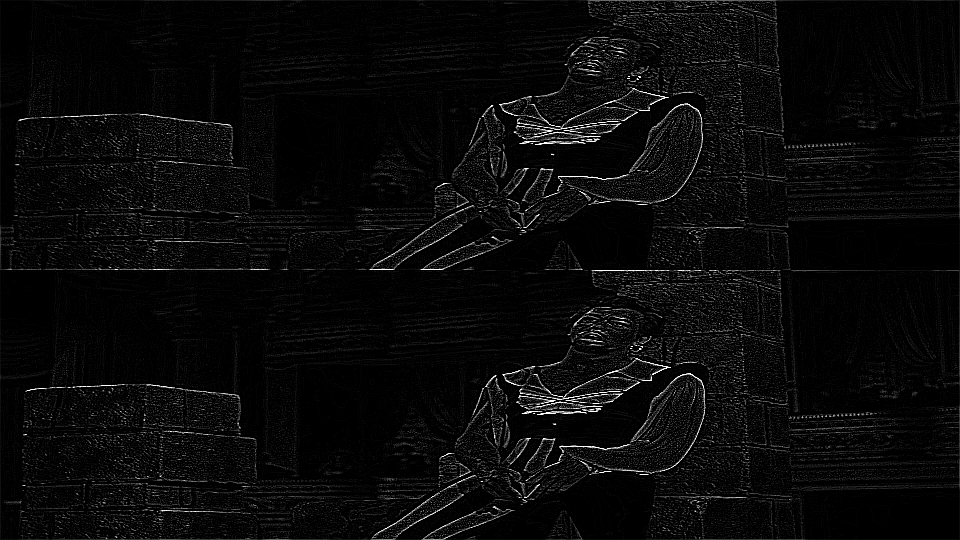You can see the line, right? It's incredibly unlikely that a random still from a movie would have a line in exactly that position - especially over several frames.

I think this is what sometimes causes my TV to make mistakes. When I play a split-screen game like Portal 2, the TV occasionally switched to 3D mode erroneously.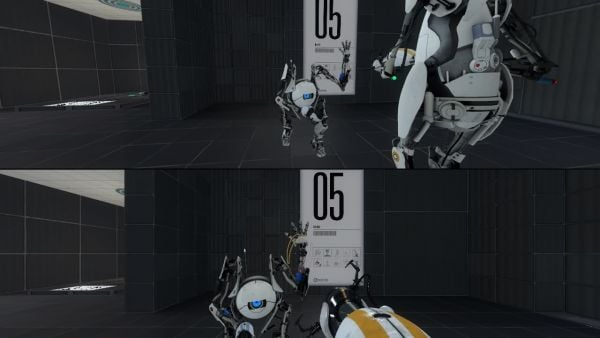## Detecting

But... how does a computer "see" that line? Especially when it isn't continuous?

We know where the line should be - either exactly halfway up or across - so we can look just at the specific pixels in those locations.

First, let's convert the image to monochrome. This means we just need to look at strong contenders for edges: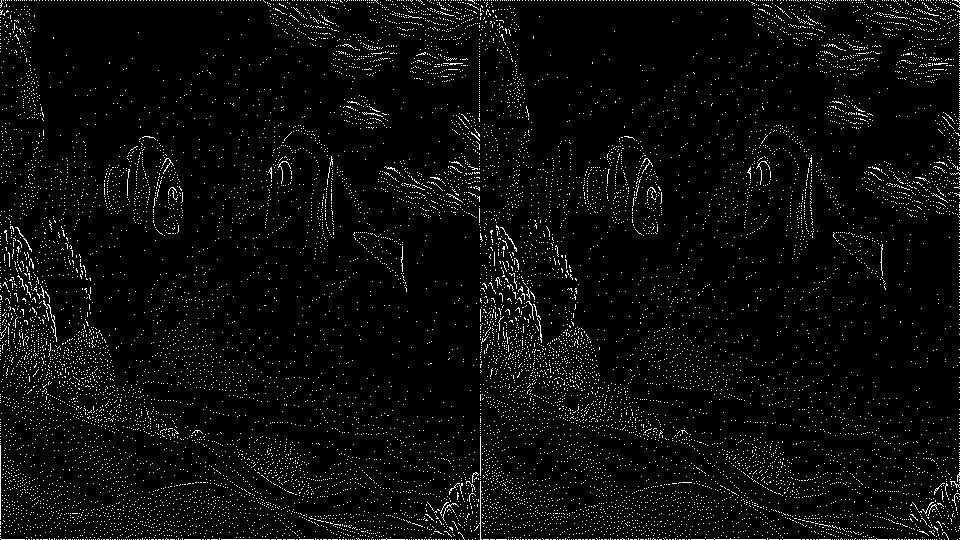Converted with:

``````Image.open("Finding_Nemo_01.png").filter(ImageFilter.FIND_EDGES).convert("1").show()
``````

We could count how many white pixels there are - but that's unreliable. Look at how noisy the image is.

Can we improve it? Sure! We need to turn of dithering when we convert it to mono.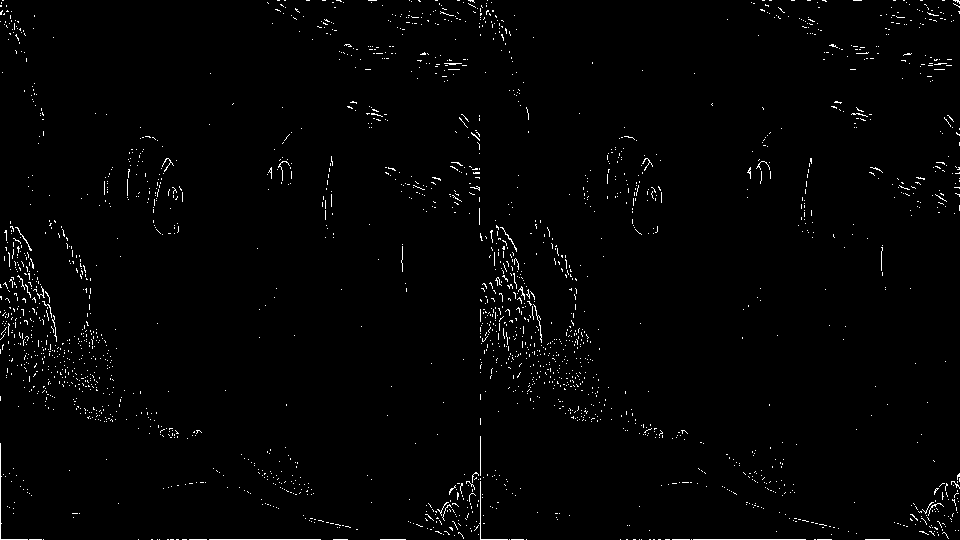``````Image.open("Finding_Nemo_01.png").filter(ImageFilter.FIND_EDGES).convert("1", dither=Image.NONE).show()
``````

We can also shrink the image. This will bring some of the lines closer together, and make it slightly quicker to count the continuous white pixels.

``````filename = "samples/Finding_Nemo_01.png"
image         = Image.open(filename)
width, height = image.size

small         = image.resize((int(width/2), int(height/2)))
width, height = small.size

greyscale = small.convert("L")
edges     = greyscale.filter(ImageFilter.FIND_EDGES)
mono      = edges.convert("1", dither = Image.NONE)

mono.show()
``````

### Find the line

Converting a Pillow Image to an array is easy:

``````import numpy as np
pixels = np.asarray(mono)
``````

But there's something annoying/confusing lurking there:

``````np.asarray(mono).shape
(1080, 1920)
``````

Yup! It rotates the image around 90 degrees. No idea why! We'll need to convert it to check the vertical line. More on that later.

When converting to a black and white image (note - not greyscale) the arrays are filled with bools. That is `True` and `False`.

To grab the horizontal line, we look halfway through the array.

``````ou_data   = pixels[int(height/2)]
``````

To grab the vertical line, we rotate the image, then look halfway through the array

``````pixels    = (np.asarray(mono.rotate(-90, expand=True)))
sbs_data  = pixels[int(width/2)]
``````

There are now two ways to determine if a line is present.

### Total number of pixels

This is the easy way. As you have seen from the images above, there are relatively few white pixels in any given column or row.

``````print("SBS total: " + str(np.sum(sbs_data)))
print("SBS mean: "  + str(np.mean(sbs_data)))
``````

If more than, say, 25% of the pixels are lit up, we can assume that there is a straight line and this is a 3D image.

### Continuous pixels

Some images are very noisy, so another strategy we can use is to count how many continuous white pixels there are along the centre horizontal line and along the centre vertical line.

I'm sure there's some fancy library for Run Length Encoding, but this simple loop is all we need.

``````def longest_line(boolean_array):
counter = 0
biggest = 0
for i in boolean_array:
if i == True:
counter += 1
if counter > biggest:
biggest = counter
if i == False:
counter = 0

return biggest
``````

## Results

Running on the SBS image

``````OU total:   15
OU mean:     2%
OU Length:   2
SBS total: 159
SBS mean:   29%
SBS Length: 59
``````

Running on the OU image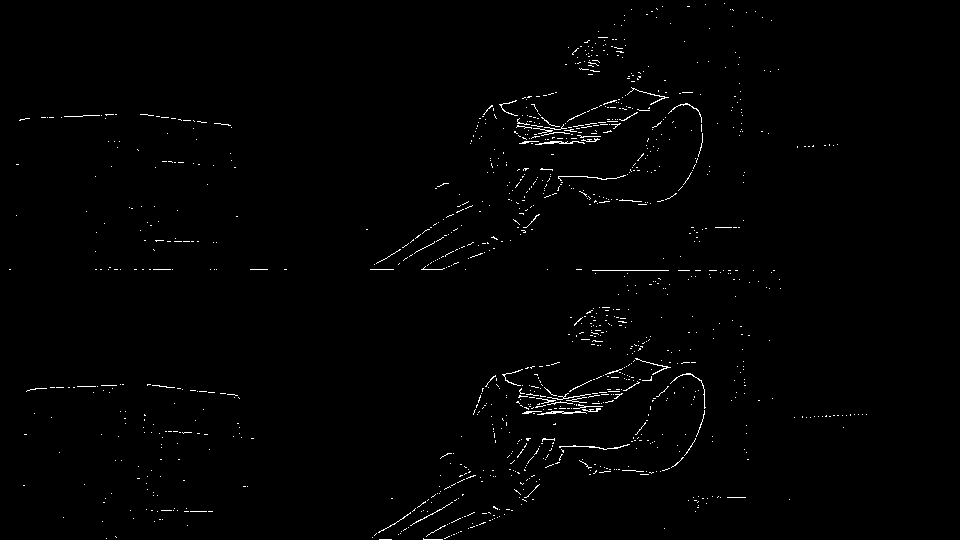``````OU total:  113
OU mean:    12%
OU Length:  77
SBS total:   9
SBS mean:    2%
SBS Length:  3
``````

## Certainty

Based on my unscientific sampling, if more than 10% of the sampled line is continuous, that's a good indication that there is a split, and this is a still from a 3D movie.

In a video we can sample every frame and if, for example, more than 5 seconds worth of frames have a line - assume that it is a 3D film.

## Image Similarity

The above techniques don't work for every 3D image though.

You will have noticed that both halves of the image are substantially similar.

There are many complex ways to detect image similarity. The simplest is calculating the Mean Square Error

``````def mse(imageA, imageB):
err = np.sum((imageA.astype("float") - imageB.astype("float")) ** 2)
err /= float(imageA.shape * imageA.shape)
return err
``````

We can split the image both horizontally and vertically, then see which pair has the smallest error.

``````from PIL import Image
import numpy as np

original = Image.open("Finding_Nemo_01.png").convert('RGB')

#   # Get the dimensions of the image
width, height = original.size

#   # Split into left and right halves.  The left eye sees the right image.
right  = original.crop( (0,       0, width/2, height))
left   = original.crop( (width/2, 0, width,   height))

#   # Over/Under. Split into top and bottom halves. The right eye sees the top image.
top    = original.crop( (0,        0, width, height/2))
bottom = original.crop( (0, height/2, width,   height))

#   # Calculate the similarity of the left/right & top/bottom.
left_right_similarity = mse(np.array(right), np.array(left))
top_bottom_similarity = mse(np.array(top),   np.array(bottom))

if (top_bottom_similarity < left_right_similarity):
#   # This is an Over/Under image
print("Over-Under image detected")
else:
print("Side-By-Side image detected")
``````

A more robust way is

``````pairs = zip(right.getdata(), left.getdata())
dif = sum(abs(c1-c2) for p1,p2 in pairs for c1,c2 in zip(p1,p2))
ncomponents = right.size * left.size * 3
print ("Difference (percentage):" + str((dif / 255.0 * 100) / ncomponents))
``````

With both, the lower the difference, the greater the similarity.

## Speed

On my crappy laptop, the basic line detection algorithm takes less than a third of a second - a quarter of second is spent reading the image from disk.

To split the image and compare it takes a similarly short amount of time. With sufficient hardware and code optimisation, 3D detection can be done in real time.

## Conclusion

I don't know that's how my TV detects 3D, but I suspect it uses a similar process. A combination of edge detection and similarity comparison is a cheap and easy way to detect 3D features.

## Putting it all together

``````from PIL import Image
from PIL import ImageFilter
import numpy as np

filename = "Finding_Nemo_01.png"

def longest_line(boolean_array):
counter = 0
biggest = 0
for i in boolean_array:
if i == True:
counter += 1
if counter > biggest:
biggest = counter
if i == False:
counter = 0

return biggest

def mse(imageA, imageB):
err = np.sum((imageA.astype("float") - imageB.astype("float")) ** 2)
err /= float(imageA.shape * imageA.shape)
return err

def difference(imageA, imageB):
pairs = zip(imageA.getdata(), imageB.getdata())
dif = sum(abs(c1-c2) for p1,p2 in pairs for c1,c2 in zip(p1,p2))
ncomponents = imageA.size * imageB.size * 3
return ((dif / 255.0 * 100) / ncomponents)

image         = Image.open(filename).convert('RGB')
width, height = image.size

small         = image.resize((int(width/2), int(height/2)))
width, height = small.size

greyscale = small.convert("L")
edges     = greyscale.filter(ImageFilter.FIND_EDGES)
mono      = edges.convert("1", dither = Image.NONE)

mono.show()

pixels    = np.asarray(mono)
ou_data   = pixels[int(height/2)]
ou_length = longest_line(ou_data)

print("OU total: " + str(np.sum(ou_data)))
print("OU mean: "  + str(np.mean(ou_data)))
print("OU Length: "+ str(ou_length))

pixels    = (np.asarray(mono.rotate(-90, expand=True)))
sbs_data  = pixels[int(width/2)]
sbs_length= longest_line(sbs_data)

print("SBS total: " + str(np.sum(sbs_data)))
print("SBS mean: "  + str(np.mean(sbs_data)))
print("SBS Length: "+ str(sbs_length))

width, height = image.size
#   # Over/Under. Split into top and bottom halves. The right eye sees the top image.
top    = image.crop( (0,        0, width, height/2))
bottom = image.crop( (0, height/2, width,   height))
#   # Calculate the difference of the top/bottom
top_bottom_mse = mse(np.array(top),   np.array(bottom))
print("Top/Bottom: " + str(top_bottom_mse))
print ("Difference (percentage):" + str(difference(top,bottom)))

#   # Split into left and right halves.  The left eye sees the right image.
right  = image.crop( (0,       0, width/2, height))
left   = image.crop( (width/2, 0, width,   height))

#   # Calculate the difference of the left/right.
left_right_mse = mse(np.array(right), np.array(left))
print("Left/Right: " + str(left_right_mse))
print ("Difference (percentage):" + str(difference(right,left)))
``````